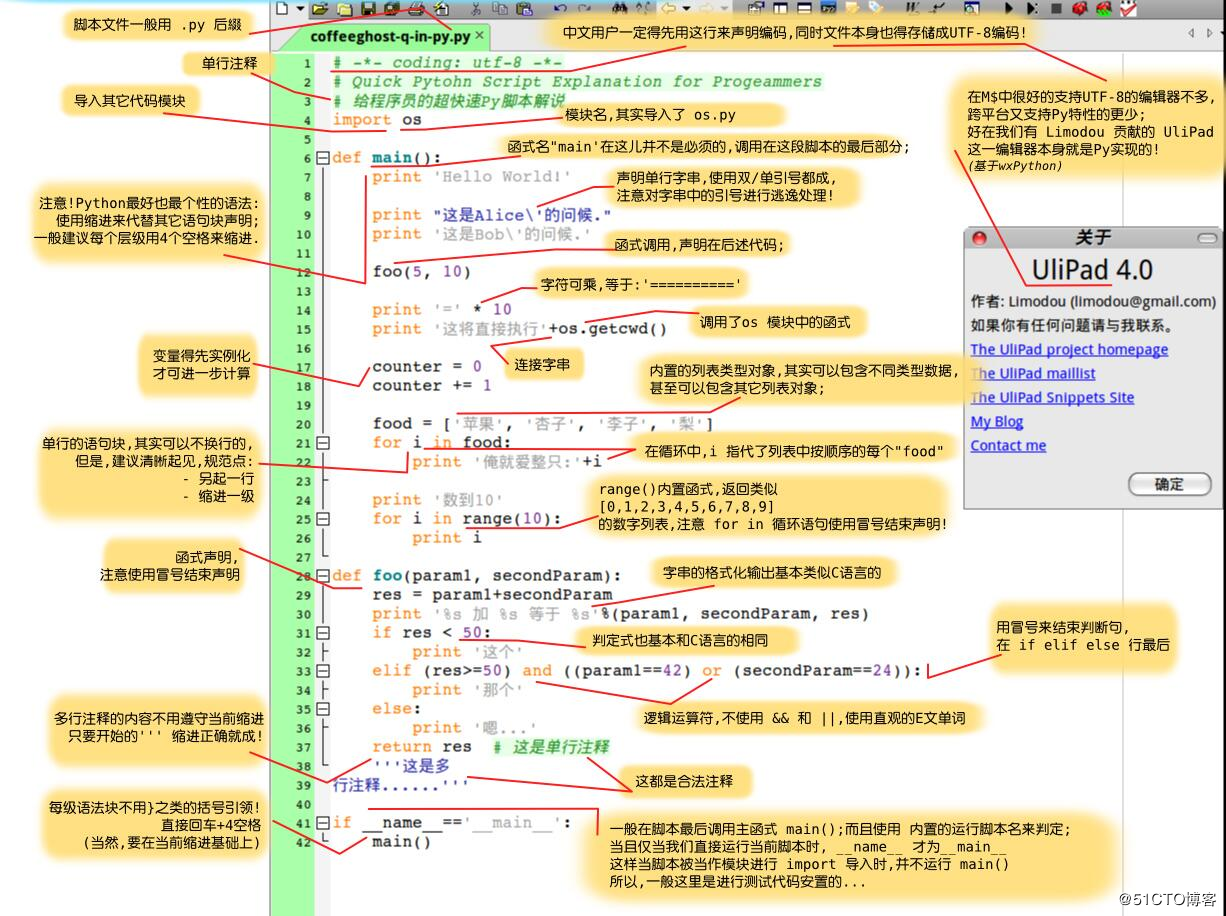# 一张图认识Python（附基本语法总结）Python基础语法总结：

1.Python标识符

Python 中的标识符是区分大小写的。

2.Python有五个标准的数据类型

Numbers（数字）
String（字符串）
List（列表）
Tuple（元组）
Dictionary（字典）
Python支持四种不同的数字类型：

int（有符号整型）
long（长整型[也可以代表八进制和十六进制]）
float（浮点型）
complex（复数）
python的字串列表有2种取值顺序:

List（列表） 是 Python 中使用最频繁的数据类型。

3.Python数据类型转换

int(x [,base])

long(x [,base] )

float(x)

complex(real [,imag])

str(x)

repr(x)

eval(str)

tuple(s)

list(s)

set(s)

dict(d)

frozenset(s)

chr(x)

unichr(x)

ord(x)

hex(x)

oct(x)

4.Python 运算符

python算术运算符

/ 除 - x除以y b / a 输出结果 2
% 取模 - 返回除法的余数 b % a 输出结果 0

// 取整除 - 返回商的整数部分 9//2 输出结果 4 , 9.0//2.0 输出结果 4.0
①：python比较运算符

== 等于 - 比较对象是否相等 (a == b) 返回 False。
!= 不等于 - 比较两个对象是否不相等 (a != b) 返回 true.
<> 不等于 - 比较两个对象是否不相等 (a <> b) 返回 true。这个运算符类似 != 。

< 小于 - 返回x是否小于y。所有比较运算符返回1表示真，返回0表示假。这分别与特殊的变量True和False等价。注意，这些变量名的大写。 (a < b) 返回 true。
= 大于等于 - 返回x是否大于等于y。 (a >= b) 返回 False。
<= 小于等于 - 返回x是否小于等于y。 (a <= b) 返回 true。

②：Python赋值运算符

= 简单的赋值运算符 c = a + b 将 a + b 的运算结果赋值为 c
+= 加法赋值运算符 c += a 等效于 c = c + a
-= 减法赋值运算符 c -= a 等效于 c = c - a
= 乘法赋值运算符 c = a 等效于 c = c * a
/= 除法赋值运算符 c /= a 等效于 c = c / a
%= 取模赋值运算符 c %= a 等效于 c = c % a
= 幂赋值运算符 c = a 等效于 c = c ** a
//= 取整除赋值运算符 c //= a 等效于 c = c // a

③：Python位运算符

a = 0011 1100
b = 0000 1101
a&b = 0000 1100
a|b = 0011 1101
a^b = 0011 0001
~a = 1100 0011

& 按位与运算符：参与运算的两个值,如果两个相应位都为1,则该位的结果为1,否则为0 (a & b) 输出结果 12 ，二进制解释： 0000 1100
| 按位或运算符：只要对应的二个二进位有一个为1时，结果位就为1。 (a | b) 输出结果 61 ，二进制解释： 0011 1101
^ 按位异或运算符：当两对应的二进位相异时，结果为1 (a ^ b) 输出结果 49 ，二进制解释： 0011 0001
~ 按位取反运算符：对数据的每个二进制位取反,即把1变为0,把0变为1 (~a ) 输出结果 -61 ，二进制解释： 1100 0011， 在一个有符号二进制数的补码形式。
<< 左移动运算符：运算数的各二进位全部左移若干位，由”<<”右边的数指定移动的位数，高位丢弃，低位补0。 a << 2 输出结果 240 ，二进制解释： 1111 0000

④：Python逻辑运算符

Python语言支持逻辑运算符，以下假设变量 a 为 10, b为 20:

and x and y 布尔”与” - 如果 x 为 False，x and y 返回 False，否则它返回 y 的计算值。 (a and b) 返回 20。
or x or y 布尔”或” - 如果 x 是非 0，它返回 x 的值，否则它返回 y 的计算值。 (a or b) 返回 10。
not not x 布尔”非” - 如果 x 为 True，返回 False 。如果 x 为 False，它返回 True。 not(a and b) 返回 False

⑤：Python成员运算符

in 如果在指定的序列中找到值返回 True，否则返回 False。 x 在 y 序列中 , 如果 x 在 y 序列中返回 True。
not in 如果在指定的序列中没有找到值返回 True，否则返回 False。 x 不在 y 序列中 , 如果 x 不在 y 序列中返回 True。

⑥：Python身份运算符

is is 是判断两个标识符是不是引用自一个对象 x is y, 类似 id(x) == id(y) , 如果引用的是同一个对象则返回 True，否则返回 False
is not is not 是判断两个标识符是不是引用自不同对象 x is not y ， 类似 id(a) != id(b)。如果引用的不是同一个对象则返回结果 True，否则返回 False。

is 用于判断两个变量引用对象是否为同一个， == 用于判断引用变量的值是否相等。

Python高级安全编程

Python实战应用开发

Python 信息安全工具开发
---------------------